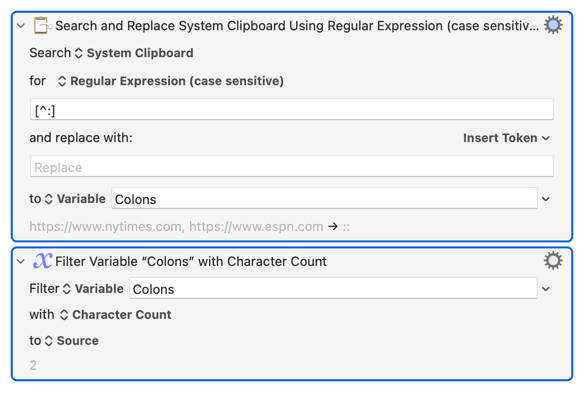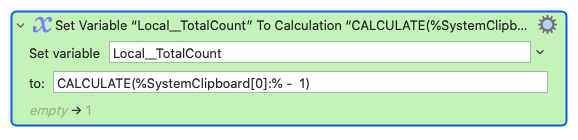# How to count the number of occurrences of a character in system clipboard text, and then set that count to a variable?

How would I count the number of occurrences of a character – specifically, the ":" character – in the current system clipboard text, and then set that count to a variable?

For example, if the system clipboard text is "https://www.nytimes.com, https://www.espn.com", the count would be "2", and that would be set to a variable.

Hi!
Here's one aproach:

Number of colons in System Clipboard Macro (v10.2)

2 Likes

Here is another possibility. Search and Replace everything that isn't a colon and remove it, and then count the remaining characters with the Filter action.2 Likes

And to put it another way, the count will always be:

one less than the number of parts resulting from splitting on that token

So, in a variant of @Alexander's approach:

(starting with the tally at -1, and incrementing on each For Each loop through the parts)

or by script in an Execute JavaScript for Automation action:

Expand disclosure triangle to view JS source
``````(() => {
"use strict";

// How many instances of particular character
// in a given text ?

const main = () => {
const kmVar = kmValue(kmInstance());

return kmVar("local_Text")
.split(new RegExp(kmVar("local_Char"), "u"))
.length - 1;
};

// ---------------- KEYBOARD MAESTRO -----------------

// kmInstance :: () -> IO String
const kmInstance = () =>
ObjC.unwrap(
\$.NSProcessInfo.processInfo.environment
.objectForKey("KMINSTANCE")
) || "";

// kmValue :: KM Instance -> String -> IO String
const kmValue = instance =>
k => Application("Keyboard Maestro Engine")
.getvariable(k, {instance});

// --
return main();
})();

``````
2 Likes
2 Likes

Thanks, everyone! I appreciate the help. I've got this up and running now.

3 Likes

I know you've got it running, but here is a very simple way to do what you want.

It depends on understanding that the clipboard contents are, in KM terms, a variable array that uses the custom delimiter of a colon - ":". The KM wiki explains this in full here: manual:Variables [Keyboard Maestro Wiki]

Consequently counting the number of colons is simply the size of the array minus one like this:Here is the action for you to import:
Count Colons.kmactions (551 Bytes)

6 Likes

Thanks, @tiffle! This is good to know.

How does this CALCULATE formula work, out of curiosity?

Why does SystemClipboard have ":" towards the end?

What does the " - 1" part do?

@tiffle is making exemplary use of Keyboard Maestro Variable Arrays here.

They allow you to split a string on a delimiter, and refer to the resulting parts by numeric index.

`` is the first part, and `` stores a count of the total.

By default, KM assumes that the string is split into parts by commas,
but, as @tiffle has done here, you can specify a custom delimiter, placing it immediately after the closing `]` – hence `:` rather than plain ``

As the number of delimiters is always one less than the total number of parts resulting from the split, @tiffle has wrapped the expression in CALCULATE, to perform a numeric (rather than string) operation, subtracting one from the number of delimited string parts.

6 Likes

Thanks for the explanation, @ComplexPoint!

1 Like